# How to Calculate and Solve for Compressional or P Waves | Rock Mechanics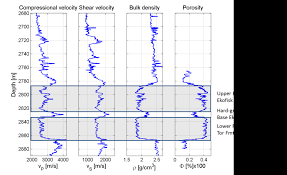The image above represents compressional or P waves.

To compute for compressional or P waves, three essential parameters are needed and these parameters are Dynamic Bulk Modulus of Elasticity (kd), Dynamic Modulus of Rigidity (Gdand Elasticity of Rock Mass (p).

The formula for calculating compressional or P waves:

vp = √[kd + 4/3Gd] / p

Where:

vp = Compressional or P Waves
kd = Dynamic Bulk Modulus of Elasticity
Gd = Dynamic Modulus of Rigidity
p = Elasticity of the Rock Mass

Let’s solve an example;
Given that the dynamic bulk modulus of elasticity is 11, the dynamic modulus of rigidity is 30 and the elasticity of the rock mass is 23. Find the compressional or P waves?

This implies that;

kd = Dynamic Bulk Modulus of Elasticity = 11
Gd = Dynamic Modulus of Rigidity = 30
p = Elasticity of the Rock Mass = 23

vp = √[kd + 4/3Gd] / p
vp = √[11 + 4/3(30)] / 23
vp = √[11 + 40] / 23
vp = √ / 23
vp = 7.141 / 23
vp = 0.310

Therefore, the compressional or P waves is 0.310.

Nickzom Calculator – The Calculator Encyclopedia is capable of calculating the compressional or P waves.

To get the answer and workings of the compressional or P waves using the Nickzom Calculator – The Calculator Encyclopedia. First, you need to obtain the app.

You can get this app via any of these means:

To get access to the professional version via web, you need to register and subscribe for NGN 1,500 per annum to have utter access to all functionalities.
You can also try the demo version via https://www.nickzom.org/calculator

Apple (Paid) – https://itunes.apple.com/us/app/nickzom-calculator/id1331162702?mt=8
Once, you have obtained the calculator encyclopedia app, proceed to the Calculator Map, then click on Geology under Add-on.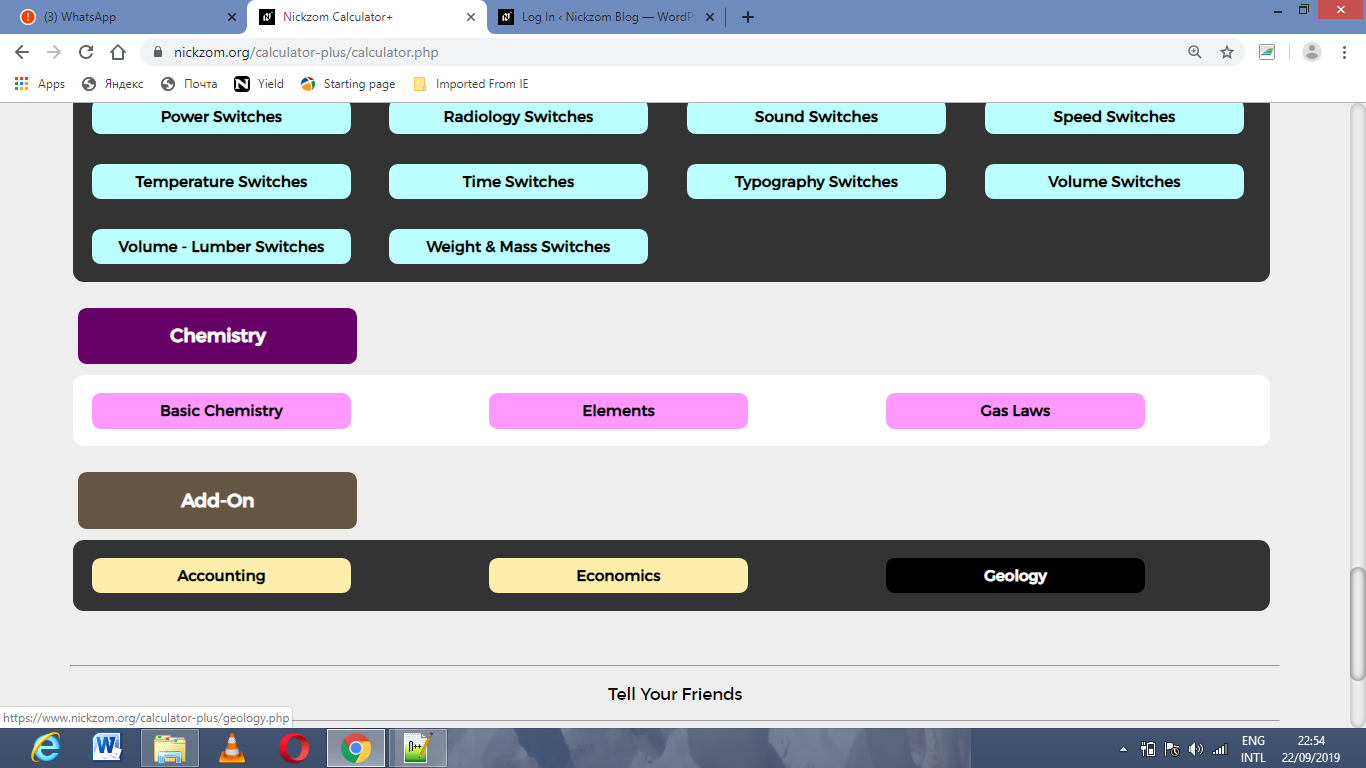Now, Click on Rock Mechanics under GeologyNow, Click on Compressional or P Waves under Rock Mechanics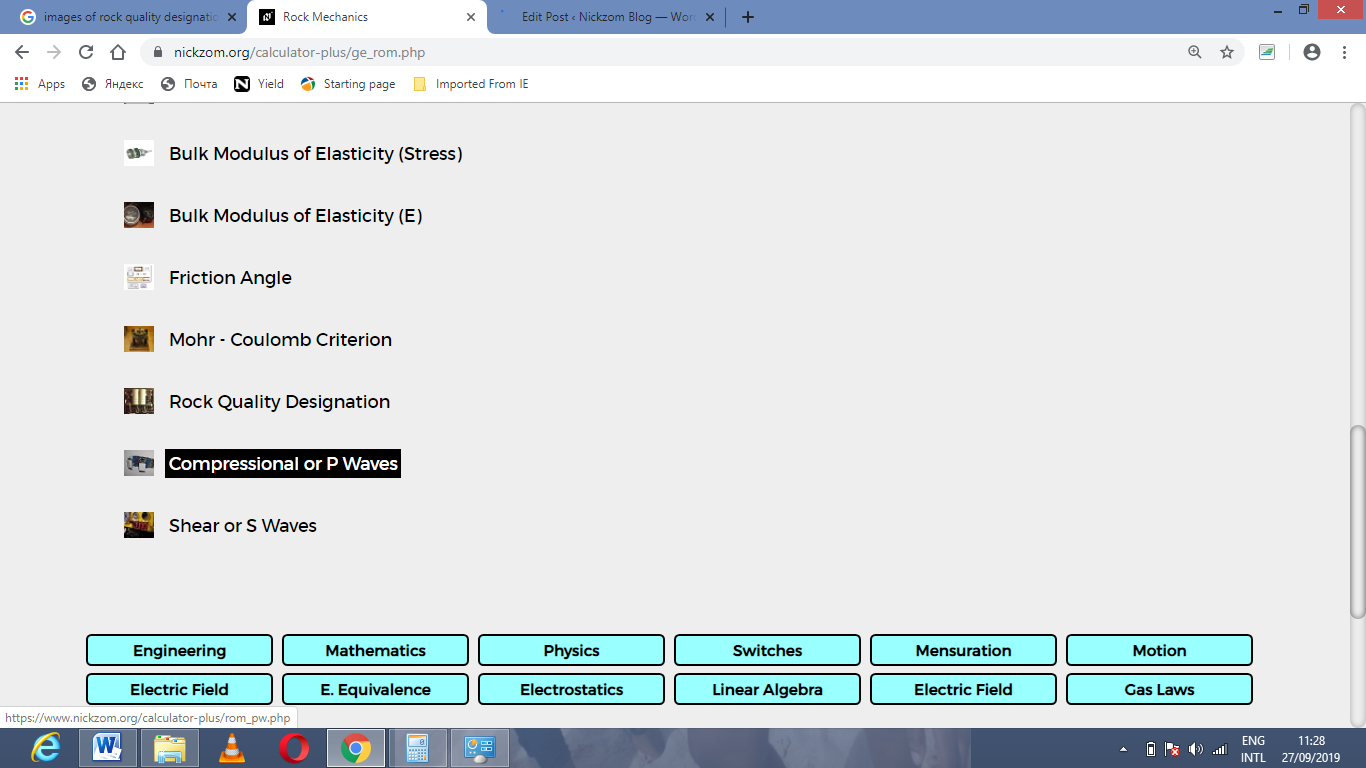The screenshot below displays the page or activity to enter your values, to get the answer for the compressional or P waves according to the respective parameters which are the Dynamic Bulk Modulus of Elasticity (kd), Dynamic Modulus of Rigidity (Gdand Elasticity of Rock Mass (p).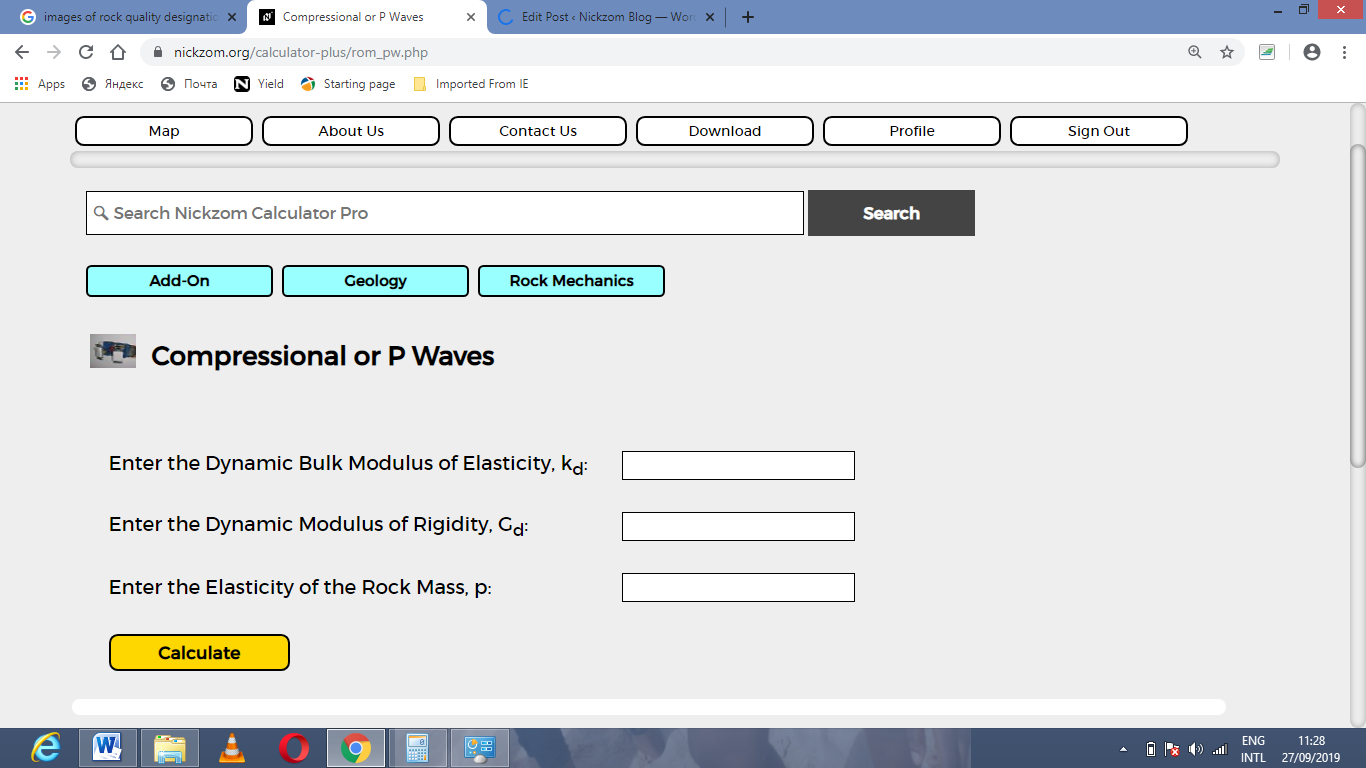Now, enter the values appropriately and accordingly for the parameters as required by the Dynamic Bulk Modulus of Elasticity (kd) is 11, Dynamic Modulus of Rigidity (Gdis 30 and Elasticity of Rock Mass (p) is 23.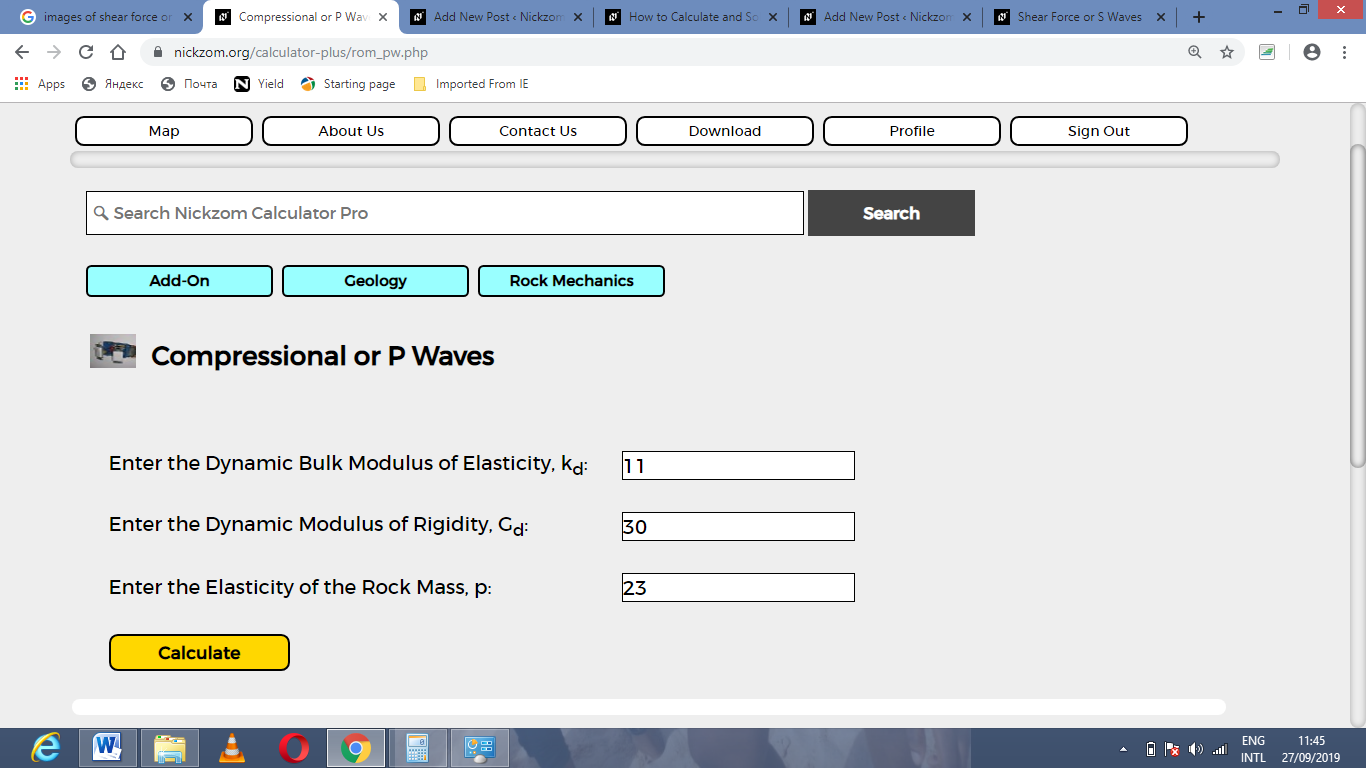Finally, Click on Calculate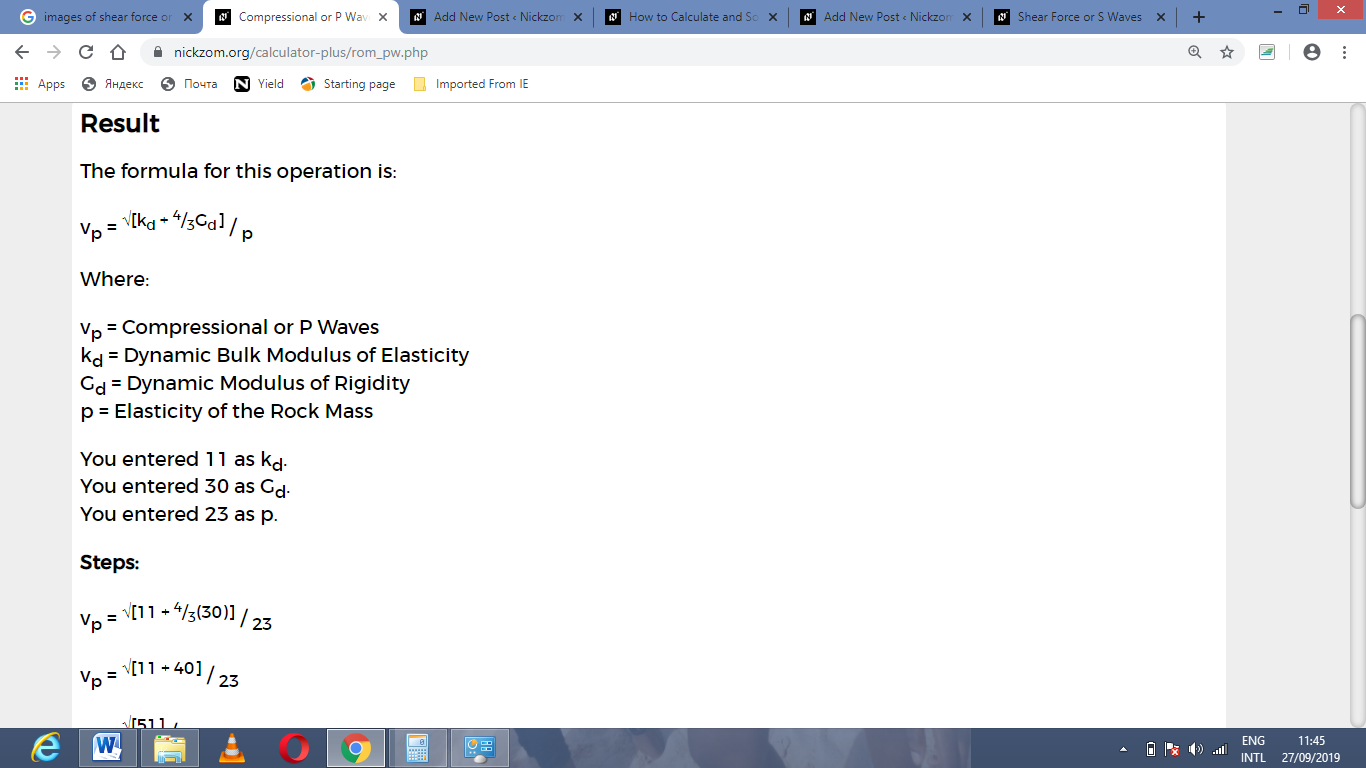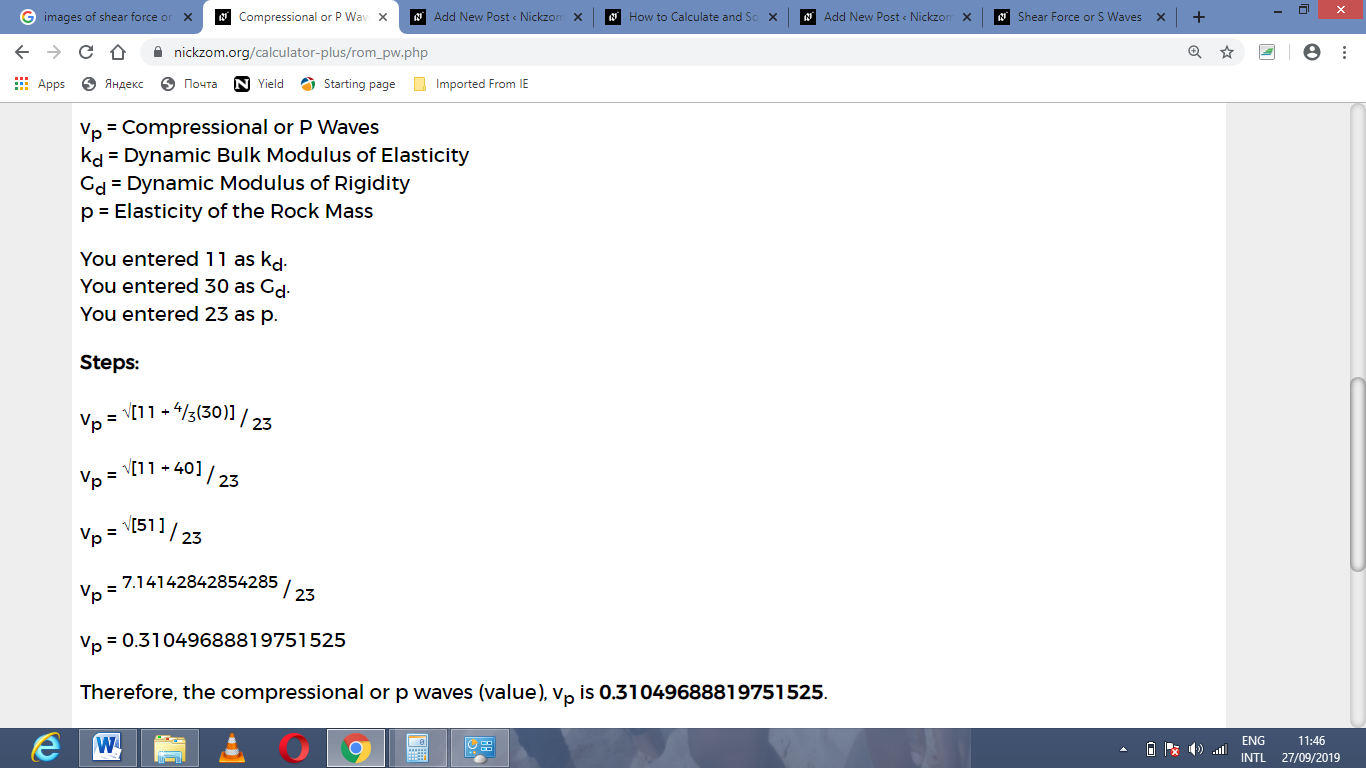As you can see from the screenshot above, Nickzom Calculator– The Calculator Encyclopedia solves for the compressional or P waves and presents the formula, workings and steps too.